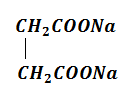• Subject:

...

• Topic:

...

${\mathrm{CH}}_{3}\mathrm{Cl}\stackrel{\mathrm{Na}}{\to }$Gas A$\underset{\mathrm{Electrolysis}}{\overset{\mathrm{Kolbe}\text{'}\mathrm{s}}{\to }}$Gas B

${\mathrm{Mg}}_{2}{\mathrm{C}}_{3}\stackrel{{\mathrm{H}}_{2}\mathrm{O}}{\to }$Gas C

Gas (A+B+C) $\underset{{\mathrm{Cu}}_{2}{\mathrm{Cl}}_{2}}{\overset{\mathrm{Ammoniacal}}{\to }}$

gas mixture  Gas X

Gas X is same is

1. Gas A + Gas B                      2. Only Gas B

3. Gas B + Gas C                      4. Only Gas A